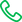0755-86570056

# 三相电表50A最大功率是多少？

2023-10-23 16:41

$$P_{max} = 3I_{max}V_{max}\cos\phi$$$$P_{max} = 3\times 50\times 380\times 0.8 = 45600 W = 45.6 kW$$